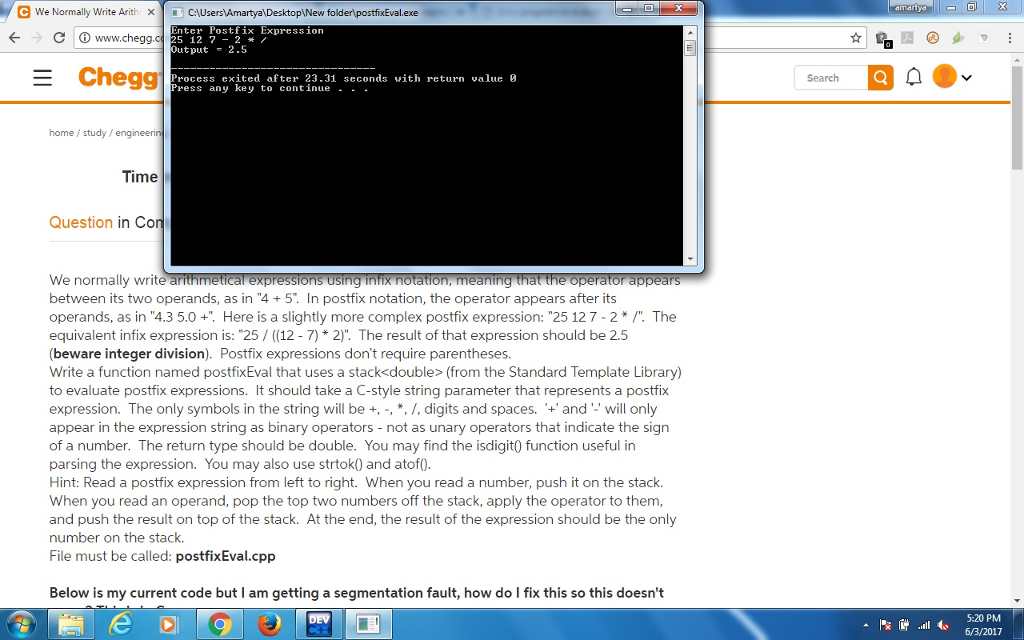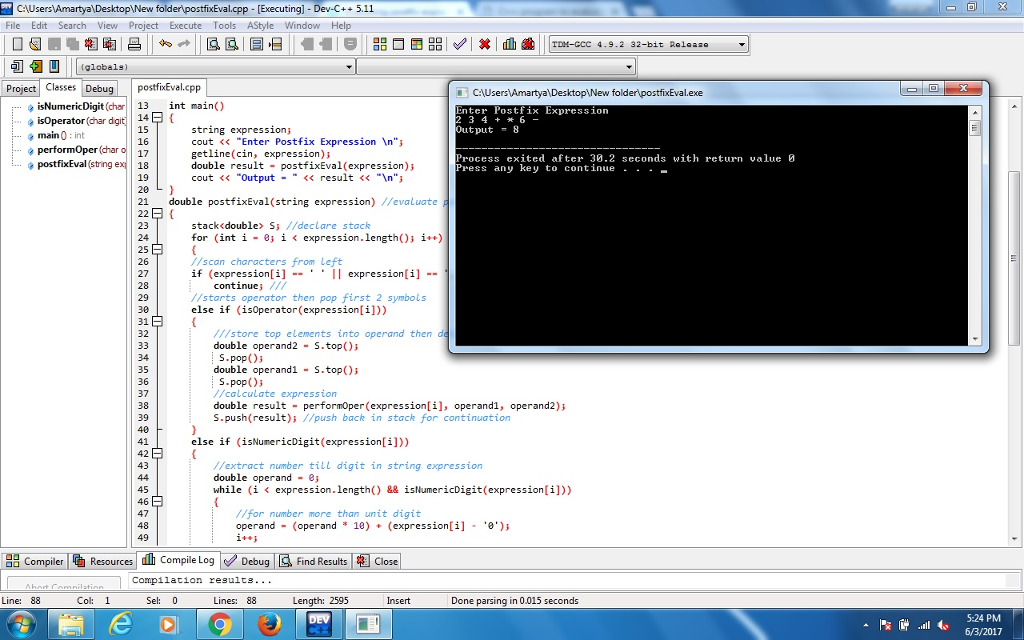# Answered! We normally write arithmetical expressions using infix notation, meaning that the operator appears between its two…

We normally write arithmetical expressions using infix notation, meaning that the operator appears between its two operands, as in “4 + 5”. In postfix notation, the operator appears after its operands, as in “4.3 5.0 +”. Here is a slightly more complex postfix expression: “25 12 7 – 2 * /”. The equivalent infix expression is: “25 / ((12 – 7) * 2)”. The result of that expression should be 2.5 (beware integer division). Postfix expressions don’t require parentheses.

Write a function named postfixEval that uses a stack<double> (from the Standard Template Library) to evaluate postfix expressions. It should take a C-style string parameter that represents a postfix expression. The only symbols in the string will be +, -, *, /, digits and spaces. ‘+’ and ‘-‘ will only appear in the expression string as binary operators – not as unary operators that indicate the sign of a number. The return type should be double. You may find the isdigit() function useful in parsing the expression. You may also use strtok() and atof().

Don't use plagiarized sources. Get Your Custom Essay on
Answered! We normally write arithmetical expressions using infix notation, meaning that the operator appears between its two…
GET AN ESSAY WRITTEN FOR YOU FROM AS LOW AS \$13/PAGE

Hint: Read a postfix expression from left to right. When you read a number, push it on the stack. When you read an operand, pop the top two numbers off the stack, apply the operator to them, and push the result on top of the stack. At the end, the result of the expression should be the only number on the stack.

File must be called: postfixEval.cpp

Below is my current code but I am getting a segmentation fault, how do I fix this so this doesn’t occur? This is in C++

#include <string>
#include <iostream>
#include <stack>

using std::cout;
using std::endl;
using std::string;
using std::cin;
using namespace std;

double postfixEval(string);

double performOper(char, double, double);

bool isOperator(char);

bool isNumericDigit(char);

int main()
{
string expression;
cout << “Enter Postfix Expression n”;
getline(cin, expression);
double result = postfixEval(expression);
cout << “Output = ” << result << “n”;
}

double postfixEval(string expression) //evaluate postfix expression
{
stack<double> S; //declare stack

for (int i = 0; i < expression.length(); i++)
{
//scan characters from left
if (expression[i] == ‘ ‘ || expression[i] == ‘,’) continue;

//starts operator then pop first 2 symbols
else if (isOperator(expression[i]))
{
///store top elements into operand then delete or pop
double operand2 = S.top(); S.pop();
double operand1 = S.top(); S.pop();
//calculate expression
double result = performOper(expression[i], operand1, operand2);

S.push(result); //push back in stack for continuation
}
else if (isNumericDigit(expression[i]))
{
//extract number till digit in string expression

double operand = 0;
while (i < expression.length() && isNumericDigit(expression[i]))
{
//for number more than unit digit
operand = (operand * 10) + (expression[i] – ‘0’);
i++;
}

//don’t want to increment i twice so out of loop
i–;

//push operand in stack
S.push(operand);
}
}
//must have one elemtn after all calculation to output
return S.top();
}

bool isNumericDigit(char digit)
{

if (digit >= ‘0’ && digit <= ‘9’)
return true;
return false;
}

bool isOperator(char digit)
{

if (digit == ‘+’ || digit == ‘-‘ || digit == ‘/’ || digit == ‘*’)
return true;
}

double performOper(char operation, double operand1, double operand2)
{
if (operation == ‘+’)
return operand1 + operand2;
else if (operation == ‘-‘)
return operand1 – operand2;
else if (operation == ‘*’)
return operand1 * operand2;
else if (operation == ‘/’)
return operand1 / operand2;

else
cout << “error”;

}

/*To fix the above posted code(in question), you need to add else condition in both isNumericDigit() and isOperator() functions, I think this would help you to run this code correctly. Then you will not get any segmentation fault. I updated your code to execute properly. Below is the final code. I have also attached output screen shots for better understanding. Hope this will help. Thank you.*/

#include <string>
#include <iostream>
#include <stack>
using std::cout;
using std::endl;
using std::string;
using std::cin;
using namespace std;
double postfixEval(string);
double performOper(char, double, double);
bool isOperator(char);
bool isNumericDigit(char);
int main()
{
string expression;
cout << “Enter Postfix Expression n”;
getline(cin, expression);
double result = postfixEval(expression);
cout << “Output = ” << result << “n”;
}
double postfixEval(string expression) //evaluate postfix expression
{
stack<double> S; //declare stack
for (int i = 0; i < expression.length(); i++)
{
//scan characters from left
if (expression[i] == ‘ ‘ || expression[i] == ‘,’)
continue; ///
//starts operator then pop first 2 symbols
else if (isOperator(expression[i]))
{
///store top elements into operand then delete or pop
double operand2 = S.top();
S.pop();
double operand1 = S.top();
S.pop();
//calculate expression
double result = performOper(expression[i], operand1, operand2);
S.push(result); //push back in stack for continuation
}
else if (isNumericDigit(expression[i]))
{
//extract number till digit in string expression
double operand = 0;
while (i < expression.length() && isNumericDigit(expression[i]))
{
//for number more than unit digit
operand = (operand * 10) + (expression[i] – ‘0’);
i++;
}
//don’t want to increment i twice so out of loop
i–;
//push operand in stack
S.push(operand);
}
}
//must have one elemtn after all calculation to output
return S.top();
}
bool isNumericDigit(char digit)
{

if (digit >= ‘0’ && digit <= ‘9’)
return true;
else /*/else condition must be explicitly written for correct output*/
return false;
}
bool isOperator(char digit)
{
if (digit == ‘+’ || digit == ‘-‘ || digit == ‘/’ || digit == ‘*’)
return true;
else /*else condition must be explicitly written for correct output*/
return false;
}
double performOper(char operation, double operand1, double operand2)
{
if (operation == ‘+’)
return operand1 + operand2;
else if (operation == ‘-‘)
return operand1 – operand2;
else if (operation == ‘*’)
return operand1 * operand2;
else if (operation == ‘/’)
return operand1 / operand2;
else
cout << “error”;
}